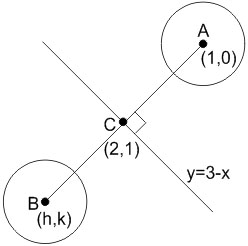Q

# I need help with - Co-ordinate geometry - JEE Main

The equation of the circle, which is the mirror image of the circle,• Option 1)• Option 2)• Option 3)• Option 4)133 Views

As learnt in

Equation of a circle -

- wherein

Circle with centre and radius .

General form of a circle -

- wherein

centre =Image of A in the line

x+y=3.

Equation of AB is

x-y=1

Coordinate of C: x+y=3

x-y=1

------------

x=2, y=1

h=3, k=2

Coordinates of B is (3,2) which is mirror image of centre (1,0) and radius is same =1

Hence equation is (x-3)2 + (y-2)2 = 1

x2+y2-6x-4y+12=0

Option 1)This option is correct.

Option 2)This option is incorrect.

Option 3)This option is incorrect.

Option 4)This option is incorrect.

Exams
Articles
Questions